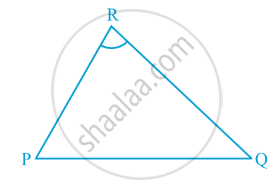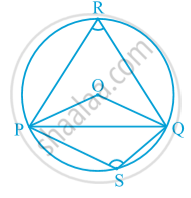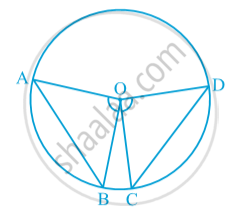# Angle Subtended by a Chord at a Point

#### notes

Take a line segment PQ and a point R not on the line containing PQ. Join PR and QR (see following  Fig.).Then  ∠ PRQ is called the angle subtended by the line segment PQ at the point R. What are angles POQ, PRQ and PSQ called in following Fig.∠ POQ is the angle subtended by the chord PQ at the centre O,
∠ PRQ and ∠ PSQ are respectively the angles subtended by PQ at points R and S on the major and minor arcs PQ.
There different chords of a circle and angles subtended by them at the centre that the longer is the chord, the bigger will be the angle subtended by it at the centre.
Draw two or more equal chords of a circle and measure the angles subtended by them at the centre.

#### theorem

Theorem: Equal chords of a circle subtend equal angles at the centre.
Proof : You are given two equal chords AB and CD of a circle with centre O (see following Fig.). You want to prove that ∠ AOB = ∠ COD.In triangles AOB and COD,
OA = OC (Radii of a circle)
OB = OD (Radii of a circle)
AB = CD (Given) Therefore, ∆ AOB ≅ ∆ COD (SSS rule) This gives ∠ AOB = ∠ COD
(Corresponding parts of congruent triangles)

Theorem:  If the angles subtended by the chords of a circle at the centre are equal, then the chords are equal.Given : ∠AOB = ∠ POQ
To prove : AB = PQ
Proof: In triangles AOB and POQ,
∠AOB = ∠POQ    (Given)
OA =OP   (Radii of same circle)
OB = OQ (Radii of same circle)
∆ AOB ≅ ∆ POQ   (SAS congruence rule)
AB = PQ (CPCT)
Hence , the theorem is proved.

If you would like to contribute notes or other learning material, please submit them using the button below.

### Shaalaa.com

Angle Subtended by a Chord at a Point [00:11:22]
S
0%